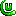﻿ Unreal Software - Thread: What is math.pi used for?

# ForumCS2DScriptsWhat is math.pi used for?

#What is math.pi used for?

2 replies
Goto Page1User
OfflineHello guys again,
I came up with a math question, I would like to know everything about
math.pi
and how to use it in lua? Probably could some of you give me some examples of using it, please? As far as i know it has a fair connection with
,
math.sin
,
math.cos
and
math.tan
.
edited 1×, last 22.03.20 08:04:46 pm
DC
Offlinemath.pi is basically a constant value. The value of pi (3.14159...)
https://en.wikipedia.org/wiki/Pi

It's required for all sorts of trigonometric calculations. Including sin/cos/tan which require an angle in radians in Lua. pi is equal to an angle of 180°. pi/2 is equal to 90° etc.

math.deg converts an angle in radians to an angle in degrees.

e.g.
math.sin(math.pi)

is equal to
OfflineThanksDC1